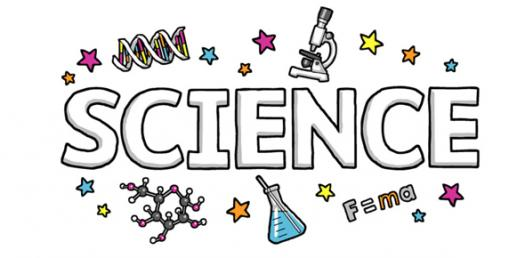# End Of Year Science

28 Questions | Total Attempts: 154SettingsThe TEKS exams are designed to cover the whole year and that being said there is a lot that one can forget if they do not practice some of the topics. Below is a 5th grade end of year science test that will enable you to pass the upcoming test. Give it a shot and all the best!

• 1.
Which of the following is an educated guess?
• A.

Problem

• B.

Hypothesis

• C.

Conclusion

• D.

Testing

• 2.
How many variables are you allowed to have in an investigation?
• A.

4

• B.

3

• C.

2

• D.

1

• 3.
Anything that has mass and takes up space is called -
• A.

Matter

• B.

Evaporation

• C.

Cycle

• D.

Weight

• 4.
Which two types of energy is released from a fire?
• A.

Electrical and light

• B.

Light and mechanical

• C.

Light and heat

• D.

Heat and sound

• 5.
Ms. Gustavus started her car on a cold moring.  When she turned on the heater in the car, all the cars windows fogged up.  Why did this happen?
• A.

It was precipitating outside

• B.

The car was insulated

• C.

Condensation was gathering on the windows

• D.

None of the above

• 6.
Ms. Gustavus mixed iron fillings and sand together.  What is the best way to seperate the two substances?
• A.

Use a filter

• B.

Use a funnel

• C.

• D.

Use a magnet

• 7.
Which of the following is the producer?
• A.

The fox

• B.

The plant

• C.

The mouse

• D.

The Sun

• 8.
If the fox is taken out of the cycle what will happen?
• A.

The would be an increase in the mouse population

• B.

There would be a decrease in the mouse population

• C.

There would be an increase in the plant population

• D.

Bears would take the place of the foxes

• 9.
Some students were exploring the properties of water.  They placed a cup with 80 mL of water into a freezer and another cup with 40 mL into another freezer.  Which of the following will be the same for both of the cups of water?
• A.

The mass of the frozen water

• B.

The weight of the frozen water

• C.

The temperature at which they freeze

• D.

The time it takes them to freeze

• 10.
Which of the following is a renewable resource?
• A.

Soil

• B.

Wind

• C.

Coal

• D.

Minerals

• 11.
Which of the following is an example of refraction?
• A.

A mirror

• B.

Black paper

• C.

Glasses

• D.

A window

• 12.
The following are all inherited traits except -
• A.

Hair color

• B.

Shape of nose

• C.

Talking

• D.

Freckles

• 13.
An insect that produces waves in the water can all so produce waves in the air.  What can these air vibration produce?
• A.

Sound

• B.

Light

• C.

Heat

• D.

Oxygen

• 14.
A student plans to make a light bulb glow by making a closed circuit.  All of the following objects can be used except -
• A.

Copper penny

• B.

Plastic comb

• C.

Metal spoon

• D.

Iron nail

• 15.
In which of the ways can volcanes build up land -
• A.

By adding heat to the Earth's surface

• B.

By adding gasses to the atmosphere

• C.

By adding lave to the Earth's surface

• D.

By adding water vapro to the atmospher

• 16.
Fossil fuels formed over along period of time because of heat and preasure were applied to -
• A.

Carbon filtered through limestone

• B.

Organisms buried in the ground

• C.

Bacteria laying on top of mud

• D.

Nitrogen mixed in the water

• 17.
Which of these causes day and night on the Earth?
• A.

The Earth rotating on its axis

• B.

The moon revolving arund the Earth

• C.

The Earth revolving around the Sun

• D.

Ms. Gustavus turning the lights on and off

• 18.
If you went to the moon which of the following would change?
• A.

• B.

• C.

• D.

• 19.
If you add sand to water what will happen to the sand?
• A.

It will dissolve

• B.

It will disapear

• C.

It float to the top

• D.

It will sink to the bottom

• 20.
How long does it take the Earth to revolve around the Sun?
• A.

24 hours

• B.

52 weeks

• C.

27 days

• D.

3 years

• 21.
Which of these can cause sharp rough mountains to become rounded over time?
• A.

Wind and rain

• B.

The Sun's rays

• C.

Light and darkness

• D.

Lava erupting from a volcano

• 22.
Which of the follwing is represented in this diagram?
• A.

Water evaporating

• B.

Coal formation

• C.

Plant cycle

• D.

The transfer of energy

• 23.
If the volume of an object is greater than its mass the object will -
• A.

Sink in water

• B.

Float in water

• C.

Dissolve in water

• D.

Nothing will happen

• 24.
Oil and water is an example of -
• A.

A solution

• B.

A reflection

• C.

A mixture

• D.

A refraction

• 25.
How can this birds adaptaion help it survive?
• A.

Swimming

• B.

Catching prey

• C.

Scratching the soil for bugs

• D.

Running

Related TopicsBack to top